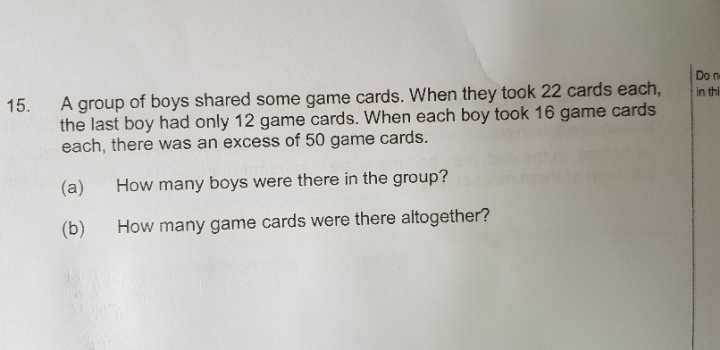# Question1 Answer

# Answer

(a)
(number of boys – 1) x 22 + 12 = (number of boys x 22) – 22 + 12 = (number of boys x 16) + 50
number of boys = (50 + 10)/(22 – 16) = 10

(b)
(10 – 1) x 22 + 12 = 210
or
10 x 16 + 50 = 210

Ans : (a) 10 boys; (b) 210 game cards.

0 Replies 0 Likes